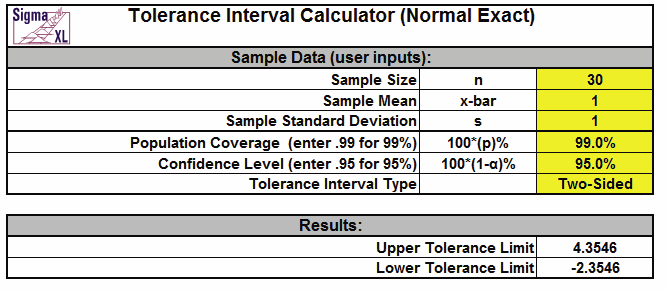Include Top

Process Capability - Tolerance Interval Calculator (Normal Exact)

Click SigmaXL > Templates & Calculators > Basic Process Capability Templates > Tolerance Interval Calculator to access the Tolerance Interval Calculator (Normal Exact). The template gives the following default example.Notes:
1. Enter summarized Sample Data, Population Coverage and Confidence Level in cells with yellow
highlight.
2. Select Tolerance Interval Type using drop-down.
3. This calculator assumes that the summary statistics come from data that is normally
distributed. Tolerance intervals are exact, one-sided uses the noncentral t distribution, two-sided
uses the noncentral chi-square distribution.
4. Due to the complexity of calculations, this template uses VBA macros rather than Excel
formulas. SigmaXL must be initialized and appear on the menu in order for this template to
function.

Web Demos

Our CTO and Co-Founder, John Noguera, regularly hosts free Web Demos featuring SigmaXL and DiscoverSim